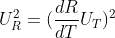## Uncertainty

Consider a resistance temperature detector (RTD) is made from platinum, and has a resistance, R, of 115 ohms, and a resistance at reference temperature (0°C) of 100 ohms. Using the below simplified Kline-McClintock equation, what is the temperature uncertainty if the resistance uncertainty is ±0.1 ohms? Note the resistance temperature coefficient for platinum is 0.004°C^(-1).Hint
$$U_{R}^{2}=(\frac{dR}{dT}U_{T})^2$$$where $$U_R$$ is the resistance uncertainty, $$dR$$ is the change in resistance, $$dT$$ is the change in temperature, and $$U_T$$ is the uncertainty in temperature. Hint 2 $$R_T=R_0[1+\alpha (T-T_0)]$$$
where $$R_T$$ is the RTD’s resistance at temperature $$T$$ , $$R_0$$ is the RTD’s resistance at the reference temperature $$T_0$$ (usually 0°C), and $$\alpha$$ is the resistance temperature coefficient.
Resistance Temperature Detector (RTD) is a device used to relate change in resistance to change in temperature. They are typically made from platinum, and have the controlling equation:
$$R_T=R_0[1+\alpha (T-T_0)]$$$where $$R_T$$ is the RTD’s resistance at temperature $$T$$ , $$R_0$$ is the RTD’s resistance at the reference temperature $$T_0$$ (usually 0°C), and $$\alpha$$ is the resistance temperature coefficient. First, solve for $$T$$ : $$115\Omega=100\Omega[1+0.004^{\circ}C^{-1}(T-0^{\circ}C)]$$$
$$1.15=1+0.004^{\circ}C^{-1}(T)$$$$$T=\frac{0.15}{0.004^{\circ}C^{-1}}=37.5^{\circ}C$$$
With $$T=37.5^{\circ}C$$ , we now know everything to solve for the temperature uncertainty. Rearranging the simplified Kline-McClintock equation:
$$U_{T}=\frac{U_{R}(dT)}{dR}$$$where $$U_R$$ is the resistance uncertainty, $$dR$$ is the change in resistance, $$dT$$ is the change in temperature, and $$U_T$$ is the uncertainty in temperature. $$U_{T}=\frac{0.1\Omega(37.5^{\circ}C-0^{\circ}C)}{115\Omega-100\Omega}=\frac{0.1(37.5^{\circ}C)}{15}=0.25^{\circ}C$$$
0.25°C